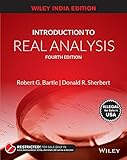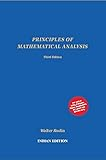# Examples and Counterexamples: Real Line with Discrete Metric/Topology

🛈 This materials is useful for Unit 1 in CSIR-NET.

Definition:$<\mathbb{R},d>$, where$d(x,y)=\begin{cases}0 & x=y\\ 1&x\ne y\end{cases}$

Prove that$d$ is a metric on$\mathbb{R}$, as an exercise.

We denote it as$\mathbb{R}_d$.

In terms of topological spaces,$<\mathbb{R},\tau>,\tau=\mathscr{P}(\mathbb{R})$, where$\mathscr{P}(\mathbb{R})$ is the power set of$\mathbb{R}$

##### Some Interesting Properties:
1. Every function is continuous in$\mathbb{R}_d$.
2. Every set is open subset of$\mathbb{R}_d$.
3. Every set is closed in$\mathbb{R}_d$.
4.$\mathbb{R}_d$ is not complete.
##### Examples of interest
1.$f(x)=$ greatest integer smaller than or equal to x is not continuous in$\mathbb{R}$ with usual metric, but is continuous in$\mathbb{R}_d$.
2.$\{a\},a\in\mathbb{R}_d$ is not open in$\mathbb{R}$, but it is open in$\mathbb{R}_d$. In fact, every set is open in$\mathbb{R}_d$
3.$\{a\},a\in\mathbb{R}_d$ is closed in$\mathbb{R}$, and in$\mathbb{R}_d$.
##### Challenges
1. Can you find an example of compact set in$\mathbb{R}_d$?
2. Can you characterize convergent sequences in$\mathbb{R}_d$?

Leave your thoughts in the comments below!

## Recommended Books for Real AnalysisIntroduction to Real Analysis by Bartle and Sherbert (4e)Principles of Mathematical Analysis by Rudin